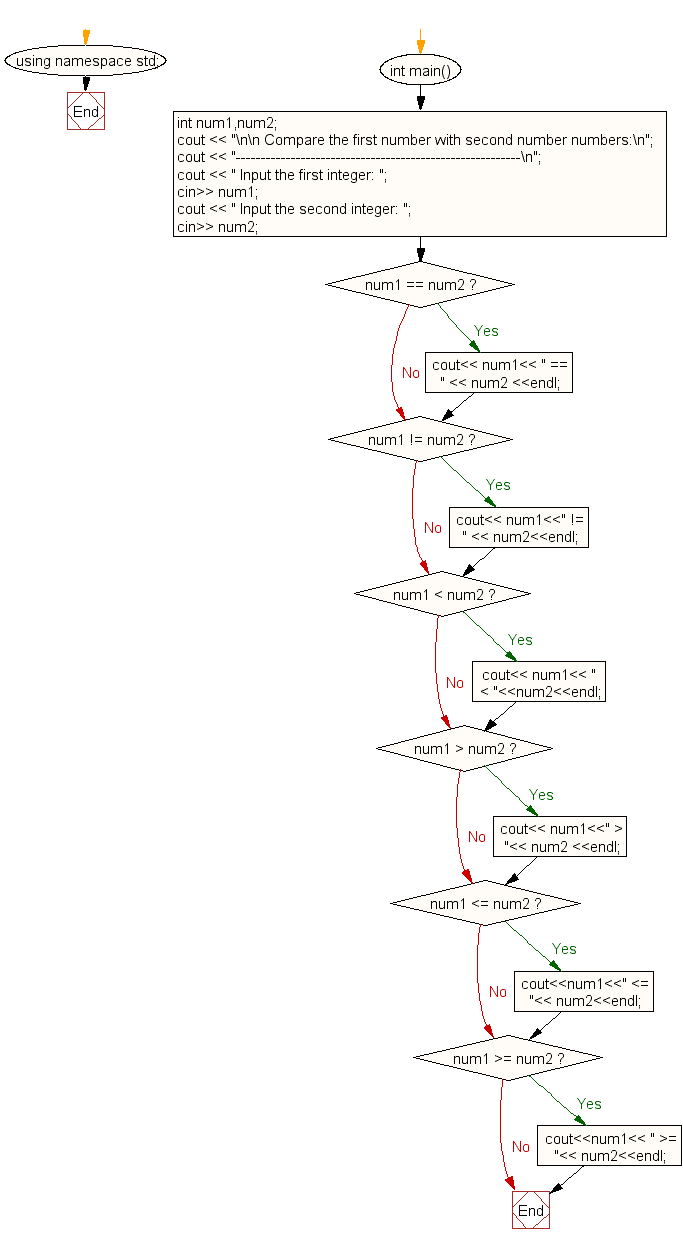﻿ C++ Exercises: Compare two numbers - w3resource

# C++ Exercises: Compare two numbers

## C++ For Loop: Exercise-82 with Solution

Write a program in C++ to compare two numbers.

Sample Solution:-

C++ Code :

``````#include <iostream> // Including the input-output stream library
using namespace std; // Using standard namespace

int main() // Main function
{
int num1, num2; // Declare two integer variables num1 and num2

// Displaying a message for the comparison of two numbers
cout << "\n\n Compare the first number with second number numbers:\n";
cout << "---------------------------------------------------------\n";

// Asking user to input the first integer
cout << " Input the first integer: ";
cin >> num1; // Taking user input for num1

// Asking user to input the second integer
cout << " Input the second integer: ";
cin >> num2; // Taking user input for num2

// Comparing and displaying if num1 is equal to num2
if (num1 == num2)
cout << num1 << " == " << num2 << endl;

// Comparing and displaying if num1 is not equal to num2
if (num1 != num2)
cout << num1 << " != " << num2 << endl;

// Comparing and displaying if num1 is less than num2
if (num1 < num2)
cout << num1 << " < " << num2 << endl;

// Comparing and displaying if num1 is greater than num2
if (num1 > num2)
cout << num1 << " > " << num2 << endl;

// Comparing and displaying if num1 is less than or equal to num2
if (num1 <= num2)
cout << num1 << " <= " << num2 << endl;

// Comparing and displaying if num1 is greater than or equal to num2
if (num1 >= num2)
cout << num1 << " >= " << num2 << endl;
}
``````

Sample Output:

``` Compare the first number with second number numbers:
---------------------------------------------------------
Input the first integer: 25
Input the second integer: 15
25 != 15
25 > 15
25 >= 15
```

Flowchart:C++ Code Editor: